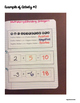# Multiplying and Dividing Integers ActivitiesSubject
Resource Type
File Type

PDF

(1 MB|23 pages)
Product Rating
4.0
(3 Ratings)
Standards
Also included in:
1. A complete 1-year Interactive Math Notebook Bundle for seventh grade. Students create and make math activities in their notebooks. All the 7th grade Math Standards are reviewed with this notebook bundle! The Bundle is 20% OFF the original price of all total interactive math activities. FEATURES:Math
\$62.00
\$50.00
Save \$12.00
2. This Middle School Interactive Math Notebooks MEGA Bundle is a COMPLETED BUNDLE covering ALL 6th, 7th, & 8th grade Common Core Standards! NO PREP needed. Just Print & GO!FEATURES:*Grade 6~Math Notebook BUNDLE {Grade 6}Math Notebook 101 GuideInteger ActivitiesFactors and Multiples ActivitiesR
\$186.00
\$146.00
Save \$40.00
3. 7th Grade Math Curriculum is a GROWING bundle that includes all Interactive Math Notebook Activities, Math Station Activities, Math notes, Math Worksheets, Math Writing Centers, & Card Sort activities for 7th Grade Math in my store.This bundle currently includes \$457.00 worth of material GROWING
\$457.00
\$250.00
Save \$207.00
• Product Description
• StandardsNEW
These interactive notebook activities are intended to help students understand how to apply and extend previous understandings of multiplication and division of fractions. Solve real-world and mathematical problems with four operations with rational numbers.Included are:

-4 different Integer activities to engage students

-Teacher facilitated activity for 60-90 minutes of classroom time

-Activities include: Vocabulary, Multiplying & Dividing with Negative Integers, Multiplying & Dividing Negative and Positive Fractions with KCF Method, and Multiplying & Dividing Negative and Positive Decimals.

-Examples of Completed Interactive Math Notebook Activities are included

***************************************************************************
Other 7th Grade NO PREP Interactive Math Notebook Activities:Middle School Interactive Math Notebook BUNDLE {Grade 7}
Middle School Interactive Math Notebook 101 Guide
Interactive Math Notebook: Rational Numbers {Grade 7}
Interactive Math Notebook: Rates and Proportionality {Grade 7}
Interactive Math Notebook: Proportions and Percents {Grade 7}
Interactive Math Notebook: Expressions & Equations {Grade 7}
Interactive Math Notebook: Inequalities {Grade 7}
Interactive Math Notebook: Modeling Geometric Figures {Grade 7}
Interactive Math Notebook: Circumference, Area, and Volume {Grade 7}
Interactive Math Notebook: Random Samples & Populations {Grade 7}
Interactive Math Notebook: Analyzing & Comparing Data {Grade 7}
Interactive Math Notebook: Experimental Probability {Grade 7}
Interactive Math Notebook: Theoretical Probability & Simulations {Grade 7}

***************************************************************************

LEAVE FEEDBACK on this product and earn 4 TPT credits to use on future purchases!

This purchase is for ONE teacher ONLY. Additional teachers must purchase their own license. You may not upload this resource to the internet in any form. If you are a coach, principal or district interested in purchasing several licenses, please contact me for a district-wide quote at kellymccowntpt@hotmail.com

For more teaching ideas visit www.kellymccown.com.
Solve real-world and mathematical problems involving the four operations with rational numbers.
Apply and extend previous understandings of multiplication and division and of fractions to multiply and divide rational numbers.
Total Pages
23 pages
N/A
Teaching Duration
90 minutes
Report this Resource to TpT
Reported resources will be reviewed by our team. Report this resource to let us know if this resource violates TpT’s content guidelines.